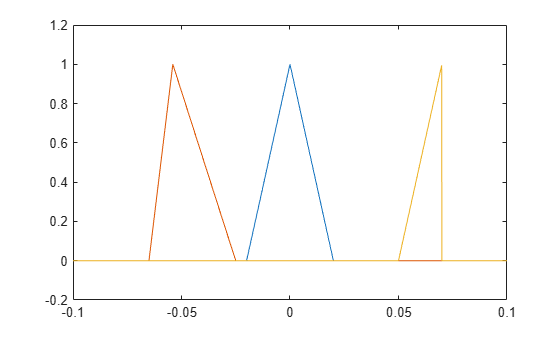# tripuls

Sampled aperiodic triangle

## Syntax

``y = tripuls(t)``
``y = tripuls(t,w,s)``

## Description

````y = tripuls(t)` returns a continuous, aperiodic, symmetric, unit-height triangular pulse at the sample times indicated in array `t`, centered about `t` = `0`.```

example

````y = tripuls(t,w,s)` generates a triangle of width `w` and skew `s`.```

## Examples

collapse all

Generate 200 ms of a symmetric triangular pulse with a sample rate of 10 kHz and a width of 40 ms.

```fs = 10e3; t = -0.1:1/fs:0.1; w = 40e-3; x = tripuls(t,w);```

Generate two variations of the same pulse:

• One displaced 45 ms into the past and skewed 45% to the left.

```tpast = -45e-3; spast = -0.45; xpast = tripuls(t-tpast,w,spast);```
• One displaced 60 ms into the future, half as wide, and skewed completely to the right.

```tfutr = 60e-3; sfutr = 1; xfutr = tripuls(t-tfutr,w/2,sfutr);```

Plot the original pulse and the two copies on the same axes.

```plot(t,x,t,xpast,t,xfutr) ylim([-0.2 1.2])```## Input Arguments

collapse all

Sample times of unit triangular pulse, specified as a vector.

Data Types: `single` | `double`

Triangle width, specified as a positive number.

Data Types: `single` | `double`

Triangle skew, specified as a real number such that –1 ≤ `s` ≤ 1. When `s` is 0, the function generates a symmetric triangular pulse.

Data Types: `single` | `double`

## Output Arguments

collapse all

Triangular pulse of unit amplitude, returned as a vector.

## Version History

Introduced before R2006a